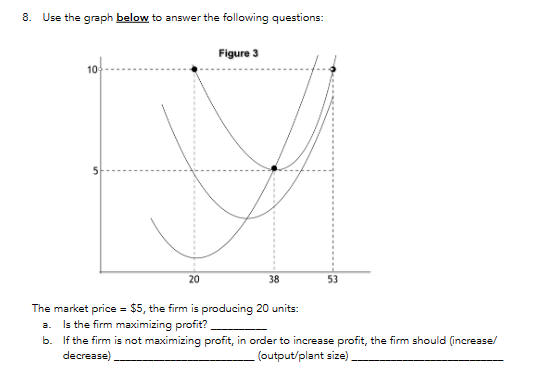# (Solved): 8. Use the graph below to answer the following questions: The market price =\$5, the firm is produc ...8. Use the graph below to answer the following questions: The market price , the firm is producing 20 units: a. Is the firm maximizing profit? b. If the firm is not maximizing profit, in order to increase profit, the firm should (increase/ decrease). (output/plant size).

We have an Answer from Expert